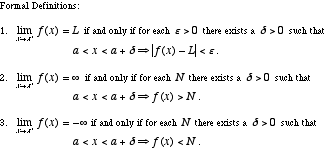index: click on a letter A B C D E F G H I J K L M N O P Q R S T U V W X Y Z A to Z index index: subject areas numbers & symbols sets, logic, proofs geometry algebra trigonometry advanced algebra & pre-calculus calculus advanced topics probability & statistics real world applications multimedia entrieswww.mathwords.com about mathwords website feedback

 Limit from the Right Limit from Above A one-sided limit which, in the example, restricts x such that x > 0. In general, a limit from the right restricts domain variable to values greater than the number the domain variable approaches. When a limit is taken from the right it is writtenor. For example,sincetends toward ∞ as x gets closer and closer to 0 from the right.See also

 this page updated 19-jul-17 Mathwords: Terms and Formulas from Algebra I to Calculus written, illustrated, and webmastered by Bruce Simmons Copyright © 2000 by Bruce Simmons All rights reserved